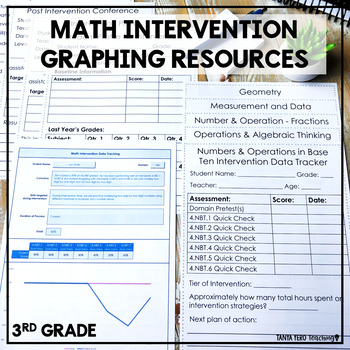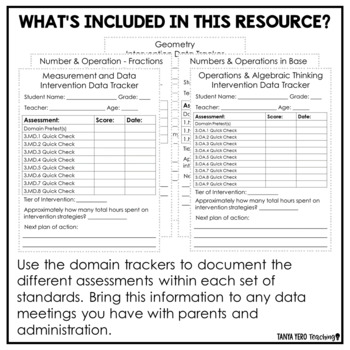# 3rd Grade RTI Documentation Forms | Math Intervention Tracking Sheets3rd - 4th, Higher Education
Subjects
Standards
Resource Type
Formats Included
• Zip
Pages
25 pages

#### Also included in

1. A comprehensive, no prep, standards-based bundle of RTI 3rd grade math intervention resources. This research-based intervention bundle includes tools for building a systematic, data-driven intervention process. With pre/post-tests (for each domain) for executing screening, quick checks for progress
\$28.00
\$36.00
Save \$8.00
2. A comprehensive, no prep, standards-based bundle of RTI math intervention resources for grades 3-5. This research-based intervention bundle includes tools for building a systematic, data-driven intervention process.With pre/post-tests (for each domain) for executing screening, quick checks for progr
\$99.00
\$127.50
Save \$28.50
3. A comprehensive, no prep, standards-based bundle of RTI math intervention resources for grades K-8. This research-based intervention bundle includes tools for building a systematic, data-driven intervention process.With pre/post-tests (for each domain) for executing screening, quick checks for progr
\$313.00
\$395.00
Save \$82.00

### Description

Everything you need to track student progress and keep you RTI efforts organized!

This file includes printable graphs and recording sheets for every step of the math intervention process. This resource pairs perfectly with our 3rd Math Intervention Bundle.

Use these forms to track RTI progress and provide documentation for parents and administration. There is a breakdown of how to use each form and tips and suggestions for filling each sheet out. There is also a digital option for tracking the intervention process provided in an Excel spreadsheet. Check out the preview to see more!

Total Pages
25 pages
Does not apply
Teaching Duration
1 Year
Report this Resource to TpT
Reported resources will be reviewed by our team. Report this resource to let us know if this resource violates TpT’s content guidelines.

### Standards

to see state-specific standards (only available in the US).
Interpret products of whole numbers, e.g., interpret 5 × 7 as the total number of objects in 5 groups of 7 objects each. For example, describe a context in which a total number of objects can be expressed as 5 × 7.
Interpret whole-number quotients of whole numbers, e.g., interpret 56 ÷ 8 as the number of objects in each share when 56 objects are partitioned equally into 8 shares, or as a number of shares when 56 objects are partitioned into equal shares of 8 objects each. For example, describe a context in which a number of shares or a number of groups can be expressed as 56 ÷ 8.
Use multiplication and division within 100 to solve word problems in situations involving equal groups, arrays, and measurement quantities, e.g., by using drawings and equations with a symbol for the unknown number to represent the problem.
Determine the unknown whole number in a multiplication or division equation relating three whole numbers. For example, determine the unknown number that makes the equation true in each of the equations 8 × ? = 48, 5 = __ ÷ 3, 6 × 6 = ?.
Apply properties of operations as strategies to multiply and divide. Examples: If 6 × 4 = 24 is known, then 4 × 6 = 24 is also known. (Commutative property of multiplication.) 3 × 5 × 2 can be found by 3 × 5 = 15, then 15 × 2 = 30, or by 5 × 2 = 10, then 3 × 10 = 30. (Associative property of multiplication.) Knowing that 8 × 5 = 40 and 8 × 2 = 16, one can find 8 × 7 as 8 × (5 + 2) = (8 × 5) + (8 × 2) = 40 + 16 = 56. (Distributive property.)